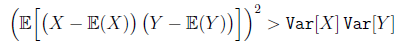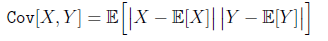# GATE | GATE CS 2021 | Set 1 | Question 45

• Difficulty Level : Hard
• Last Updated : 24 May, 2021

Consider the two statements.

S1: There exist random variables X and Y such thatS2: For all random variables X andWhich one of the following choices is correct?
(A) Both S1 and S2 are true
(B) S1 is true, but S2 is false
(C) S1 is false, but S2 is true
(D) Both S1 and S2 are false

Explanation: Theorem : Square of Covariance is Less Than or Equal to Product of Variances.

Let X and Y be random variables.
Let the variances of X and Y exist and be finite.
Then:
(cov(X,Y))2 ≤ var(X)var(Y)
where cov(X,Y) denotes the covariance of X and Y.

Proof :
We have, by the definition of variance, that both:

E((X−E(X))2)
and:
E((Y−E(Y))2)
exist and are finite.

Therefore:

(cov(X,Y))2 = (E((X−E(X))(Y−E(Y))))2
Definition of Covariance
(cov(X,Y))2 ≤ E((X−E(X))2)E((Y−E(Y))2)
Square of Expectation of Product is Less Than or Equal to Product of Expectation of Squares
(cov(X,Y))2 = var(X)var(Y)
Definition of Variance.

Hence, both given statement (S1) and (S2) are false.

Quiz of this Question

My Personal Notes arrow_drop_up
Recommended Articles
Page :